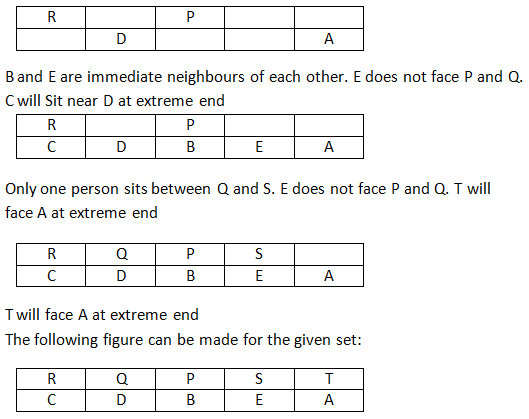### logical reasoning questions for clat: logical reasoning questions for clat that you should solve for improvement

Practising a lot of logical reasoning questions for clat exam will surely give an upper edge to your preparation. Learning concepts and then implementing them through the medium of logical reasoning questions for clat will help you get a stronger hold on concepts. Following the past year exam trends, following is a compilation of the topics on which logical reasoning questions for clat exam are based:
• Statement Conclusion/ Assumption
• Pairing
• Data Interpretation
• Inference based question
• Calendar
• Directions
• Para-jumbles
• Puzzles
• Seating Arrangement
• Logical Sequences
• Number Test
• Verbal Reasoning
• Family/ Blood Relation
• Ranking & Order
• Coding Decoding
• Syllogisms

Directions for the question set:
Study the following information to answer the given questions:
Ten people are sitting in two parallel rows containing five people each, in such a way that there is an equal distance between adjacent persons. In row — 1 P, Q, R, S and T are seated and all of them are facing South. In row — 2 A, B, C, D and E are seated and all of them are facing North. Therefore, in the given seating arrangement each member seated in a row faces another member of the other row.
D sits third to the left of A. P faces immediate neighbour of D. R sits second to the right of P. Only one person sits between Q and S. B and E are immediate neighbours of each other. E does not face P and Q.

Question 1: How many persons are seated between Q and T?
(a) None
(b) One
(c) Two
(d) Three
(e) Cannot be determined

Question 2: Four of the following five are alike in a certain way and thus form a group. Which is the one that does not belong to that group?
(a)R
(b) S
(c) C
(d) T
(e) A

Question 3: Who amongst the following represents the people sitting exactly in the middle of the rows?
(a) P, E
(b) S, D
(c) S, A
(d) A, R
(e) P, B

Question 4: Which of the following is true regarding B?
(a) A and C are immediate neighbours of B
(b) B sits at one of the extreme ends of the line
(c) Q faces B
(d) T is an immediate neighbour of the person facing B
(e) D sits to the immediate left of B

Question 5: Four of the following five are alike in a certain way and thus-form a group. Which is the one that does not belong to that group?
(a) T-E
(b) Q-C
(c) S – B
(d) R – A
(e) P – D

Question 6: Who amongst the following faces S?
(a) A
(b) B
(c) C
(d) D
(e) E

### Answers and Explanations: Click the down arrow to expand

Common Solution for the set: D sits third to the left of A. P faces immediate neighbour of D. R sits second to the right of P.Answer 1: (c) There are 2 people between Q and T. Correct option is (C).

Answer 2: (b) All are at the end except S Correct option is (b)

Answer 3: (e) P and B sit in the middle. Correct option is (e).

Answer 4: (e) D sits to the immediate left of B. Correct option is (e).

Answer 5: (d) R – A does not follow the general trend of other options. Others follow the trend: The first person is opposite the person who is to the right of the 2nd person. Correct option is (d).

Answer 6: (e) E faces S Correct option is (e).

Extra tips for logical reasoning questions for clat:
• While solving logical reasoning questions for clat exam, make sure you first attempt the questions that you know and do not get stuck on any question.
• Use approximation wherever possible and do not get caught in lengthy calculations.
• Refer past year logical reasoning questions for clat exam from various topics to cover all the question types.